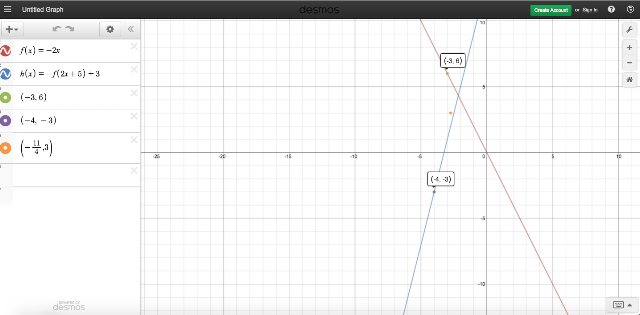## Thursday, December 17, 2015

### Desmos to the Rescue

It's midterm time here already, and I am tutoring a student in Pre-Calculus Honors. He texted me this question from his quiz last night when he was studying:

Given that the point (-3, 6) is on the graph of f(x), where does this point move to
given that h(x) = -f(2x+5) +3?

He had a wrong answer, and the teacher corrected it to (-11/4, -3).

We took horizontal stretches PLUS translations out of the curriculum except with trig (which we teach in a different way, using quarter- and half-periods) and except for in Pre-Calculus Honors. So I was trying to remember my way of doing it.

I factor out the 2 to get: h(x) = -f(2(x + 5/2)+ 3, and then make a rule. (x,y) --> (1/2x - 5/2, -y+3). Essentially, after explaining and using Desmos for discovery, students see that what happens on the outside of the function happens to "y" in the correct order (i.e., multiply by a -1 and then add 3--or reflect over the x-axis and shift up 1--see the "blue"), and what happens to the "x" happens oppositely (i.e., divide x by 2, then subtract 5/2--see "purple)"). So I took (-3, 6) and substituted into my rule (1/2x - 5/2, -y+3) and got the transformed point (-4, -3). Not the x-value my excellent colleague got.

So of course, I think I'm wrong. I text two other colleagues in the department. They both get x = -11/4. I am really confused at this point. I remember another way a former colleague used to teach the horizontal transformation. He would say, let x' be the transformed value, and let x be the original value. so 2x' + 5 = x and solve for x'. You get x' = (x-5)/2. This is the same thing that I got, but I just distributed the 1/2. So I'm really thinking I'm right because I got my answer two different ways. Still, for my highly esteemed colleagues to all agree on a different answer...I'm stumped. They are, too.

So out comes Desmos. The first equation I enter is f(x) = -2x, because the point (-3 6) lies on the graph. The next equation I enter is h(x) = -f(2x+5) + 3..
And now I have proof that this method is correct. The orange point is not on the transformed function, but (-4, -3) is.

So thanks, Desmos. And it's not about being right, because I would have been satisfied knowing I was wrong, too. It's about being able to prove how to do it, so that we can collaborate on the answer and determine, perhaps the best way to teach it. And to know that at 10 pm, we can sleep peacefully and not stay stumped :)

Even though I was right, I find this one of the most difficult topics to teach. Students can understand it momentarily, but they really can't recall it after the test. If anyone has any ways that work for them, please leave a response at the bottom of this post. I would love to hear it!